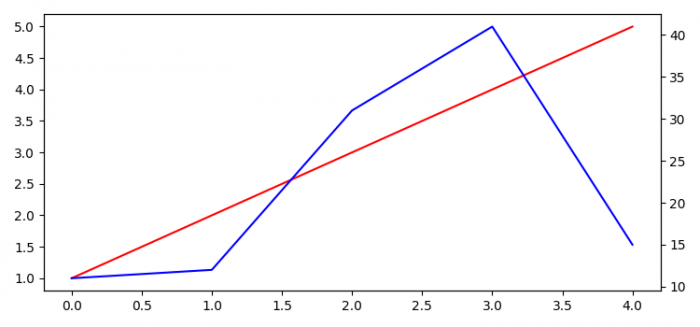# How to get multiple overlapping plots with independent scaling in Matplotlib?

To get multiple overlapping plots with independent scaling in Matplotlib, we can take the following steps −

• Set the figure size and adjust the padding between and around the subplots.

• Create a figure and a set of subplots.

• Plot a list of data points using plot() method on a seperate Y-axis and overlapping X-axis.

• Create a twin Axes sharing the X-axis.

• Plot a list of data points using plot() method on a seperate Y-axis and overlapping X-axis.

• To display the figure, use show() method.

## Example

import matplotlib.pyplot as plt

plt.rcParams["figure.figsize"] = [7.50, 3.50]
plt.rcParams["figure.autolayout"] = True

fig, ax1 = plt.subplots()
ax1.plot([1, 2, 3, 4, 5], color='red')

ax2 = ax1.twinx()
ax2.plot([11, 12, 31, 41, 15], color='blue')

plt.show()

## Output